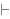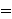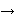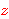Intuitionistic Logic Explorer < Previous   Next > Nearby theorems Mirrors  >  Home  >  ILE Home  >  Th. List  >  equtrr Unicode version

Theorem equtrr 1638
 Description: A transitive law for equality. Lemma L17 in [Megill] p. 446 (p. 14 of the preprint). (Contributed by NM, 23-Aug-1993.)
Assertion
Ref Expression
equtrrProof of Theorem equtrr
StepHypRef Expression
1 equtr 1637 . 221com12 30 1Colors of variables: wff set class Syntax hints:wi 4 This theorem was proved from axioms:  ax-1 5  ax-2 6  ax-mp 7  ax-ia1 104  ax-gen 1379  ax-ie2 1424  ax-8 1436  ax-17 1460  ax-i9 1464 This theorem depends on definitions:  df-bi 115 This theorem is referenced by:  equtr2  1639  equequ2  1641
 Copyright terms: Public domain W3C validator